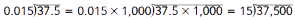Toggle Accessibility Tools

# 6-5 Decimal Ops - Concepts and Explanations

## Choosing an Operation and Estimating

Students encounter various situations involving decimals. They decide which operations to use to find a solution. Students estimate to choose operations and check their work.

### Examples

Chakara makes a rectangular tablecloth that is 3.5 meters long and 1.5 meters wide. What is the area of the tablecloth?

The dimensions are about 4 meters by about 2 meters. To find the approximate area, multiply 4 x 2. To find the exact area, multiply 3.5 x 1.5.

## Addition and Subtraction of Decimals

Decimals As Fractions Write the decimals as fractions. Find common denominators and add or subtract the fractions. Then express the answer as a decimal.

### Example

Zeke buys cider for \$1.97 and pretzels for \$.89. What is the total cost?

Written as fractions with denominator 100, the cost is 197/100 + 89/100 or 286/100, or 2.86. This is comparable to thinking of the cost in pennies and writing the sum in dollars.

Place Value Interpretation Students analyze the meaning of each digit of a number. They see that they must compute with digits that occupy like places when adding or subtracting numbers.

### Example

To find the difference 3.725 - 0.41, subtract thousandths from thousandths (0.005 - 0.000), hundredths from hundredths (0.02 - 0.01), tenths from tenths (0.7 - 0.4), and ones from ones (3 - 0).

## Multiplication of Decimals

Decimals As Fractions Write the decimals as fractions. Multiply the fractions. Then write the answer as a decimal. The number of decimal places in the factors relates to the number of decimal places in the answer.

### Example

Find the product 0.3 x 2.3. As fractions, this is 3/10 x 2 3/10 = 3/10 x 23/10; the product is 69/100, or 0.69, The denominator of the fraction tells the place value of the decimal.

Place Value Interpretation Students find patterns in sets of problems to see why counting decimal places makes sense.

### Example

Find the product 0.25 x 0.31. Use the fact that 25 x 31 = 775. Tenths x tenths results in hundredths in the product, so 2.5 x 3.1 = 7.75. Tenths * hundredths results in thousandths, so 2.5 x 0.31 = 0.775. Hundredths x hundredths results in ten-thousandths, so 0.25 x 0.31 = 0.0775.

## Division of Decimals

Decimals As Fractions Express decimals as fractions. Find common denominators. Then divide the numerators.

### Example

Find the quotient 3.25 , 0.5.

Rewrite the expression as 325/100 ÷ 5/10 = 325/100 ÷ 50/100. This is the same as 325 ÷ 50, which is 6 1/2 or 6.5.

Place Value Interpretation Write an equivalent problem: multiply the dividend and the divisor by the same power of ten until both are whole numbers.

### Example

This approach explains why moving decimal points works.## Decimal Forms of Rational Numbers

Finite (Terminating) Decimals Rational numbers with decimal forms that “end” are finite decimals. The simplified fraction form has only 2s or 5s in the prime factorization of the denominator.

### Example

1/2 = 0.5; 3/4 = 0.75; 1/8 = 0.125; 12/75 = 0.16

Infinite (Repeating) Decimals Rational numbers with decimal forms that “continue forever” but repeat are infinite decimals. The simplified fraction form has numbers other than 2 or 5 in the prime factorization of the denominator.

### Example

1/3 = 0.3333...; 2/3 = 0.6666...; 8/15 = 0.5333...; 3/7 = 0.4285714285714...

## Finding Percents

This Unit includes primarily three types of percent problems:

Find a percent of a number, based on the total and the percent rate

### Example

Jill buys a \$7.50 CD. Sales tax is 6%. How much is the tax?

1% of \$7.50 = 1/100 of \$7.50, or 0.075. So 6% of \$7.50 is 0.075 x 6, or \$.45

Find the total amount, based on the percent of the amount and the percent rate

### Example

20% of some number is \$2.50. It takes five 20,s to make 100,. 5 x \$2.50 = \$12.50, so the total bill was \$12.50.

Sam got a \$12 discount on a \$48 shirt. What percent was the discount?

Find the percent rate, based on the percent of the amount and the total.

# Example

Sam got a \$12 discount on a \$48 shirt. What percent was the discount?

There are four 12s in 48, or the percent is 1/4 of 100%, or 25%.